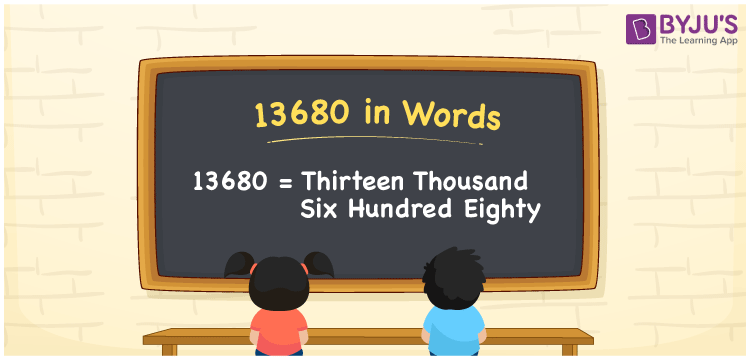# 13680 in Words

We can write 13680 in words as Thirteen thousand six hundred eighty. If you spent Rs. 13680 in a mart, you can say, “I spent Thirteen thousand six hundred eighty rupees at the mart”. Thus, we can express 13680 in word form as Thirteen thousand six hundred eighty. Let’s learn how to convert the number 13680 into words with the help of a place value chart here in this article.

 13680 in words Thirteen thousand six hundred eighty Thirteen thousand six hundred eighty in numerical form 13680

## 13680 in English Words

Generally, we use the English alphabet to write numbers in words. So, we can express 13680 in English words as “Thirteen thousand six hundred eighty”.## How to Write 13680 in Words?

In this section, you will understand how to write the number 13680 in words using a place value chart. For this, we need to make a five-column chart since 13680 is a five-digit number.

 Ten thousand Thousands Hundreds Tens Ones 1 3 6 8 0

Here, ones = 0, tens = 8, hundreds = 6, thousands = 3, ten thousand = 1

Let’s expand these digits as follows.

1 × Ten thousand + 3 × Thousand + 6 × Hundred + 8 × Ten + 0 × One

= 1 × 10000 + 3 × 1000 + 6 × 100 + 8 × 10 + 0 × 1

= 10000 + 3000 + 600 + 80

= Ten Thousand + Three thousand + Six hundred + Eighty

= Thirteen thousand + Six hundred eighty {since ten + three = thirteen}

= Thirteen thousand six hundred eighty

13680 is a natural number that is the successor of 13679 and the predecessor of 13681.

13680 in words – Thirteen thousand six hundred eighty

Is 13680 an odd number? – No

Is 13680 an even number? – Yes

Is 13680 a prime number? – No

Is 13680 a composite number? – Yes

Is 13680 a perfect square number? – No

Is 13680 a perfect cube number? – No

## Frequently Asked Questions on 13680 in Words

Q1

### How do you write 13680 in English words?

In English words, we can write 13680 as Thirteen thousand six hundred eighty.
Q2

### How to write Rs. 13680 in words on a cheque?

On a cheque, we can write Rs. 13680 in words as “Thirteen thousand six hundred eighty rupees only”.
Q3

### What is the number name for 13680?

The number name for 13680 is given as Thirteen thousand six hundred eighty.
Test your Knowledge on 13680 in Words